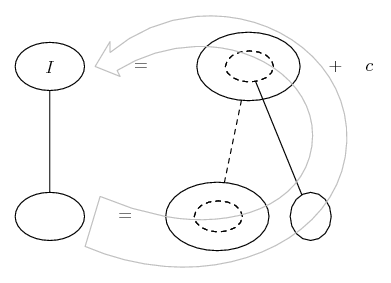# Thread: u substitution problem

1. ## u substitution problem

integrate:

[(1/3)x^3]*(1+x^4)^(1/2)

u = 1 + x^4
du = 4x^3dx

but i have no idea how to finish it from here any help would be greatly appreciated...i took cal 1 about a year ago and i forgot how to properly use u substitution

2.Originally Posted by maybnxtseasnintegrate:

[(1/3)x^3]*(1+x^4)^(1/2)

u = 1 + x^4
du = 4x^3dx

but i have no idea how to finish it from here any help would be greatly appreciated...i took cal 1 about a year ago and i forgot how to properly use u substitution
$\displaystyle \displaystyle \frac{1}{3} \int \frac{x^3}{\sqrt{1+x^4}} \, dx$

$\displaystyle u = 1 + x^4$

$\displaystyle du = 4x^3 \, dx$

$\displaystyle \displaystyle \frac{1}{12} \int \frac{4x^3}{\sqrt{1+x^4}} \, dx$

$\displaystyle \displaystyle \frac{1}{12} \int \frac{1}{\sqrt{u}} \, du$

can you finish?

3. how did u get x^3 in the numerator? did u missread my problem?

4.Originally Posted by maybnxtseasnintegrate:

[(1/3)x^3]*(1+x^4)^(1/2)

u = 1 + x^4
du = 4x^3dx

but i have no idea how to finish it from here any help would be greatly appreciated...i took cal 1 about a year ago and i forgot how to properly use u substitution
$\displaystyle \displaystyle\frac{1}{3}\int{x^3\sqrt{1+x^4}}\;dx$

$\displaystyle \displaystyle\ u=1+x^4\Rightarrow\frac{du}{dx}=4x^3\Rightarrow\fr ac{du}{4}=x^3dx$

$\displaystyle \displaystyle\int{x^3\sqrt{1+x^4}\;dx=\int{\sqrt{1 +x^4}\;x^3dx$

The integral becomes

$\displaystyle \displaystyle\frac{1}{12}\int{u^{\frac{1}{2}}du$

5.Originally Posted by maybnxtseasnhow did u get x^3 in the numerator? did u missread my problem?
I did ... should be $\displaystyle \displaystyle \frac{1}{3} \int x^3 \sqrt{1+x^4} \, dx$

same sub ...

$\displaystyle \displaystyle \frac{1}{12} \int 4x^3 \sqrt{1+x^4} \, dx$

$\displaystyle \displaystyle \frac{1}{12} \int \sqrt{u} \, du$

thought I saw a negative on the 1/2 exponent.

6. ive worked my problem down to the last step both of u have...now do i just integrate the simple equation with u? and then plug in for u and du? to get my final awnser?

so for example
if i integrate your last equation i get
(2/3)u^(3/2) du <---- do i just plug in (1+x^4) for u and im done?

7. Yes, as your final solution needs to be in terms of x.
Also add on the constant of integration.
The u-substitution allows a "convenient" solution.

For the calculation of definate integrals (not necessary here), you may choose to return to x,
or evaluate the definate integral using u with a change of limits to u.

8. (1/18)(1+x^4)^(3/2) du <----- do i just change du to dx or am i missing something for the final answer because that seems to easy?

9.Originally Posted by maybnxtseasn(1/18)(1+x^4)^(3/2) du <----- do i just change du to dx or am i missing something for the final answer because that seems to easy?
no "du" in the antiderivative.

the antiderivative is ...

$\displaystyle \displaystyle \frac{1}{18}(1+x^4)^{\frac{3}{2}} + C$

take the derivative to confirm.

10. \displaystyle \displaystyle \begin{aligned} \frac{1}{3}\int{x^3\left(1+x^4}\right)^{\frac{1}{2 }}\;{dx} & = \frac{1}{4(3)}\int{\left(1+x^4}\right)^{\frac{1}{2 }}(1+x^4)'\;{dx} \\& = \frac{2}{3(4)(3)}\int{\left[\left(1+x^4}\right)^{\frac{3}{2}}\right]'\;{dx} \\& = \frac{1}{18}\left(1+x^4}\right)^{\frac{3}{2}}+k.\e nd{aligned}

11. @ maybenxtseasn: The Coffee Machine's point relates (I think) to your earlier question about u-substitution and the chain rule... to which the answer was yes, the first one is only for navigating the second. So if the first is bogging down you might want to try a different navigational aid (if only to see how the u-sub should be working). Here's my version of what TCM is pointing out...To find F, integrate with respect to the dashed balloon (which is like where you integrate with respect to u)...So you have...... where (key in spoiler) ...

Spoiler:... is the chain rule. Straight continuous lines differentiate downwards (integrate up) with respect to the main variable (in this case x), and the straight dashed line similarly but with respect to the dashed balloon expression (the inner function of the composite which is subject to the chain rule).

The general drift is..._________________________________________

Don't integrate - balloontegrate!

Balloon Calculus; standard integrals, derivatives and methods

Balloon Calculus Drawing with LaTeX and Asymptote!

12.Originally Posted by maybnxtseasn(1/18)(1+x^4)^(3/2) du <----- do i just change du to dx or am i missing something for the final answer because that seems to easy?
The purpose of the u-substitution was to make it easy!

$\displaystyle \displaystyle\frac{d}{dx}\left(x^4+C\right)=4x^3\R ightarrow\ \int{d\left(x^4+C\right)}=\int{4x^3}\;dx\Rightarro w\int{4x^3}dx=x^4+C$

$\displaystyle \displaystyle\frac{d}{du}\left(u^4+C\right)=4u^3\R ightarrow\int{4u^3}du=u^4+C$

$\displaystyle \displaystyle\int{\sqrt{1+x^4}\;x^3dx=\int{\sqrt{u }}\;du$

when $\displaystyle u=1+x^4$

#### Search Tags

problem, substitution